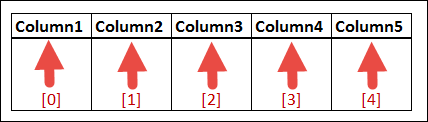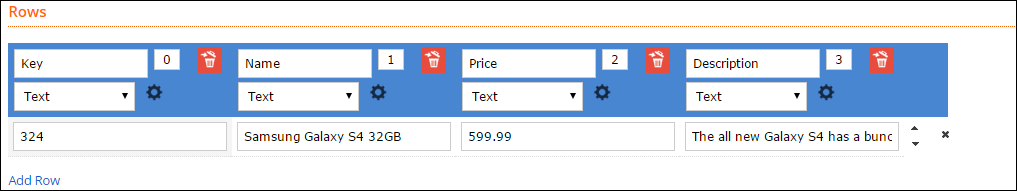The Form Designer allows you to link Choices fields to a Data Source that you have previously uploaded.

Data Sources can have as many columns as you like, and you may want to refer to the values in these columns when creating a Form.
To do so you will need to use a formula to refer to the desired column.

By default the first column in a Data Source (also known as the Value column) is always used as a field's answer.
If you want to refer to any other column's value, you need to use an index syntax - specifically you need to add square brackets around the column's index number - e.g. {{product[index]}}

Data Source column indexes always start at zero - i.e. the first column has index of 0, the second column has index 1, third column is index 2, and so on.Lets look at an example
Imagine you have a Data Source called "Products" that contains your product list, with rows such as:You create a Form named "Sales Order" which you will use to capture orders for your products.

The Form has the following fields:

• product_choice : A Choices field that is linked to the Products Data Source.
• quantity : A Number field that captures the quantity of product ordered.
• total : A Number field that will calculate the total amount by multiplying the product's price by quantity.

On the "total" field, you would define a Dynamic Value formula as follows: {{product_choice}} * {{quantity}}Note the square brackets and index value of 2 used to refer to the 3rd column (the Price) in the Products Data Source.